# Tag Archives: dates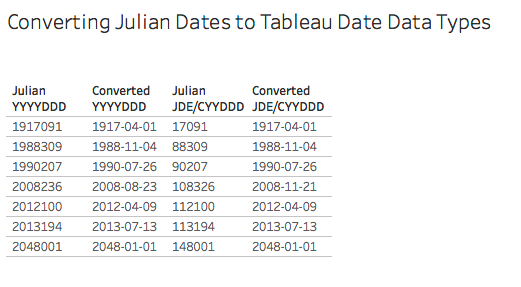# A Dating Lesson: Using Math to Get Dates (by Converting Julian Dates)

I recently had to convert some date fields formatted as Julian dates into more usable Tableau date data types (it’s not a date format that Tableau natively understands) and my web search got me a little frustrated because all the solutions I found were long formulas using string manipulation functions like LEFT(), RIGHT(), etc. That kind of solution can get the job done, but there’s something to be left desired:

String manipulation is sloooooooooooooooooooooooooooooowwww.

How slow? How about one thousand times slower (or more) than using math functions! I like my calculations to be efficient from the beginning: The practical reason is that I don’t have to spend time later going back to do performance tuning. Computers were built to do math really really fast so whenever possible I’ll use math to do my date conversions. The aesthetic reason is that there’s an elegance to creating equations that use the least number of steps, and the play reason is because math is fun!

Therefore in this post I’ll describe a faster, more elegant, and more fun way (IMO) of converting Julian dates into Tableau dates. Note that in some cases you might be able to do the conversion to a date data type inside your database, that could be even faster than the formulas I describe here.

## What is a Julian date, anyways?

There are two formats of Julian dates that I’ve run into:

• The JDE (for JD Edwards) or CYYDDD format where the DDD is the day of the year (a number from 1 to 366), YY is the two digit year, and C is the century where 0 is 1900, 1 is 2000, and so on. For example 5001 is 1905-01-01 and 117365 is 2017-12-31.
• The “modern Julian date” or YYYYDDD format where DDD is again the day of year and YYYY is the four digit year, so 2017365 would be 2017-12-31.

Now the Julian dates might be stored in raw data as strings or as numbers, either way we’re going to work with them as numbers. So if you’re starting out with Julian dates as strings then the first step is to right-click on those fields and change the data type to Number (Whole).

## Learning Three Math & Date Techniques

We can convert Julian JDE/CYYDDD dates in a single formula:

```DATE(DATEADD('day',[Julian JDE/CYYDDD] % 1000 - 1,

There are three techniques at work in this formula:

1. Extract the right-most N digits from a number using the modulo operator, for example to get the DDD value.
2. Remove the right-most N digits from a number to extract the left-most M digits from a number using the INT() function, for example to get the CYY value.
3. Using DATEADD() to add intervals to a starting date and nest for each date_part as necessary.

### Extracting the right-most N digits from a number

The slow way to get the right-most N digits from a number would be something like INT(RIGHT(STR([number]),N)). We can completely avoid the string conversion and need for RIGHT() by using some math.

The modulo operator % is a math function that returns the remainder for a whole number. So 5 % 2 returns 1, 17 % 10 returns 7, and so on. We can use this to return the right-most N digits from a number without needing to do any string manipulation by using [number] % 10^N.

In our case `[Julian JDE/CYYDDD] % 1000` will return the last 3 digits of the field corresponding to the DDD aka day of year.

A couple of other uses of the modulo function are jittering marks in a dot plot and creating groups/bins based on the right-most digits when numbers are categorical variables.

### Remove the right-most N digits from a number to extract the left-most M digits from the number

The starting way to get the left-most M digits from a number would be something like INT(LEFT(STR([number]),M)). However this gets more complicated with the JDE/CYYDDD format because depending on the date then we might want the 2 left most digits (for years in the 1900s) or the 3 left most digits (for years in the 2000s) which would lead to even more string manipulation to get the desired result, for example here’s a formula I found online for getting the year: `IF LEN([Julian JDE/CYYDDD]) = 6 AND LEFT([Julian JDE/CYYDDD], 1) = '1' THEN '20' + MID([Julian JDE/CYYDDD], 2, 2) ELSEIF LEN([Julian JDE/CYYDDD]) = 5 THEN '19' + MID([Julian JDE/CYYDDD], 1, 2) END`. All those LEN(), LEFT(), and MID() calls will be slooooww.

Thinking about this from a math standpoint what we want to do is with the JDE/CYYDDD format is to remove the DDD (the right-most N digits) and extract what’s left. The way we can do that is in two steps: 1) turn the DDD portion into a decimal so CYYDDD becomes CYY.DDD, then 2) truncate the DDD decimal portion so we’re just left with the CYY.

We can do that with the formula INT([number] / 10^N). The [number] / 10^N divides the number by the number of digits we want to remove, so 5001 becomes 5.001, 117365 becomes 117.365, and so on. Then the INT() around that truncates the decimal places and leaves us with the remaining whole number.

In our case` INT([Julian JDE/CYYDDD] / 1000)` removes the right-most three digits so what we’re left with is the century and number of years.

I regularly use this technique to build my own bins, for example INT([Sales] / 100) * 100 creates bins of 0, 100, 200, and so on.

### Using DATEADD() to add a number of intervals to a starting date

Tableau’s DATEADD() function takes three arguments:

• date_part: a value like ‘day’, ‘month’, ‘hour’, etc.
• interval: a whole number of intervals
• date: a starting date

The advantage of using DATEADD() over something like DATE([lots of string manipulation to build a string of YYYY-MM-DD or MM/DD/YYYY or DD/MM/YYYY format]) is again that we’re avoiding all that string manipulation and just doing date math, which is really math.

With the first two techniques we’ve converted the JDE/CYYDDD format to into the intervals for the day date_part and the intervals for the year date_part, so now we can use DATEADD() once for each date_part in a nested fashion. All we need is a starting date, and in this case we can take advantage of the CYY structure to figure out the appropriate starting date. This is the number of years since 1900, so to generate the year we can use:

`DATEADD('year',INT([Julian JDE/CYYDDD]/1000),#1900-01-01#)`

Then to add the DDD day of year we can use DATEADD() with a starting date of the year date we just made. We do need to subtract 1 from the DDD day of year because the first day of the year is 1, not 0.

```DATEADD('day',[Julian JDE/CYYDDD] % 1000 - 1,

## Julian JDE/CYYDDD Conversion

Tableau’s DATEADD() always returns a datetime data type, so using the above formula and wrapping the calculation in a final DATE() gives us the desired date data type:

```DATE(DATEADD('day',[Julian JDE/CYYDDD] % 1000 - 1,

Here’s a Tableau view showing the DDD and CYY calculations along with the final Converted JDE/CYYYDDD calculation: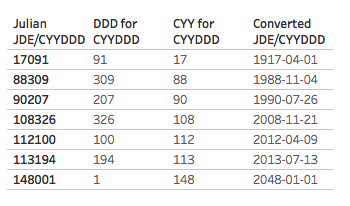## Julian YYYYDDD Conversion

The YYYYDDD conversion uses a quite similar formula, with one change:

```DATE(DATEADD('day',[Julian YYYYDDD] % 1000 - 1,

The difference is that the YYYY portion of the calculation is returning the full four digit year so we subtract 1900 from that to get a number of years to add to the 1900-01-01 start date.

Here’s a Tableau view showing the DDD and YYYY calculations along with the final Converted YYYYDDD calculation: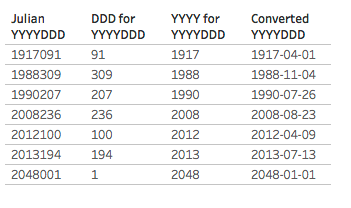## Conclusion

Math is fun. Math is fast. Math is your friend. Use math to get dates. (Date data types, that is).

Here’s a link to the converting julian dates to dates workbook on Tableau Public.

A little plug: If you like this post (and can tolerate the occasional bad pun) and want help in finding dates (in your data) please check out my company, DataBlick. We offer Tableau and Alteryx support, consulting, and training and can help you get the most out of your data!# Formatting Time Durations in Tableau

This post was updated on 24 July 2015 to include additional details on applying the custom formatting calculation to aggregates.

Here’s a quick lunchtime post on working with durations in Tableau. By duration, I mean having a result that is showing the number of seconds, minutes, hours, and/or days in the form of dd:hh:mm:ss. This isn’t quite a built-in option, there are a several ways to go about this:

• Use any duration formatting that is supported in your data source, for example by pre-computing values or using a RAWSQL function.
• Do a bunch of calculations and string manipulations to get the date to set up. I prefer to avoid these mainly because they can be over 1000x slower than numeric manipulations. If you want to see how to do this, there’s a good example on this Idea for Additional Date Time Number Formats. (If that idea is implemented and marked as Released, then you can ignore this post!)
• If the duration is less than 24 hours (86400 seconds), then you can use Tableau’s built-in date formatting. I’ll show how to do this here.
• Do some calculations and then use Tableau’s built-in number formatting. This is the brand-new solution and involves a bit of indirection.# Durations Guaranteed To Be Less Than 24 Hours: Tableau’s Date Formatting

If you know your duration is always going to be less than 24 hours (86400 seconds), , then you can take advantage of Tableau’s built-in date formatting. The first step is to get your duration in seconds, if it’s not already. For example, for looking at the duration between two dates you can use DATEDIFF(‘second’,[Start Time], [End Time]). Once you have that number of seconds, then you can use the following calculation

If the total time is less than 24 hours, then we can use the following calculation: DATETIME([Seconds]/86400). Then we can use Tableau’s date format to set the format as hh:nn:ss (in this case hh:mm:ss will also work):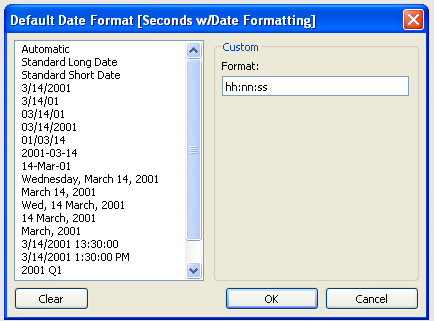Here’s a view…take a look at what happens at 86400 seconds and beyond: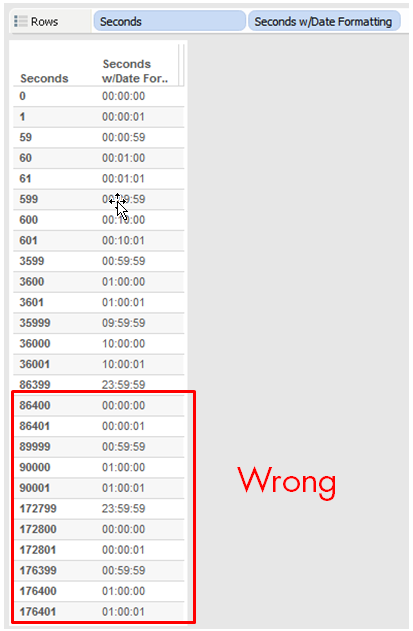Instead of showing 24 hours, the date formatting looks just at the hours, minutes, and seconds. As long as your duration is less than 86400 seconds, everything will be simple using this technique.

# Any Duration: Using Arithmetic and Number Formatting

Though Tableau’s built-in formatting is not as powerful as Excel, we can still do quite a bit. Check out Robert Mundigl’s Custom Number Formats for a pretty exhaustive list of what can be done. (He’s also got a fantastic post on String Calculations that is worth checking out.)

For example, we can set up a custom number format of 00:00, and that will perfectly format a number as a time…up to 59 seconds, that is: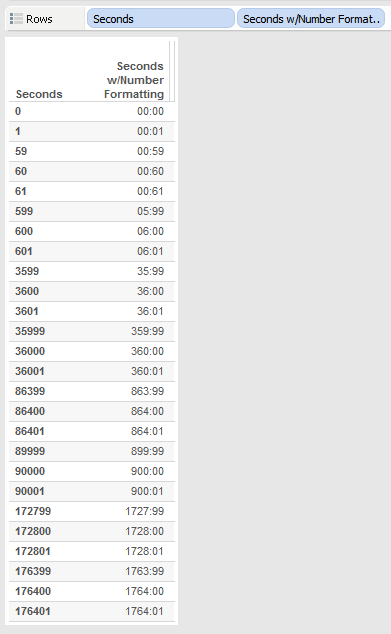However, this is a clue to the next step, which is that since we space out numbers by colons, all we need to do is get the right value into the right decimal place. So, for example, instead of 60 seconds (1 minute) being a value of 60, it has to have a value of 100 so the 00:00 formatting will make it 01:00. Here’s a formula that does just that for mm:ss values:

```//replace [Seconds] with whatever field has the number of seconds in it
//and use a custom number format of 00:00:00:00
//(drop the first 0 to get rid of leading 0's for minutes)
IIF([Seconds] % 60 == 60,0,[Seconds] % 60)// seconds
+ INT([Seconds]/60) * 100 //minutes```
In this formula, [Seconds] is a record-level field. In order for the calculation to work accurately in some situations (such as using it with Subtotals and Grand Totals) you’ll need to change [Seconds] to SUM([Seconds]) or some already-aggregated calculated field. –added 2014-12-22 per notes in the comments.

The formula uses the % (modulo) function to divide the number of seconds by 60 to get the remainder, the IIF statement is there to deal with what happens when the number of seconds is divisible by 60, the result of that is the number of seconds, then the number of minutes *100 is added to that. Here’s a view: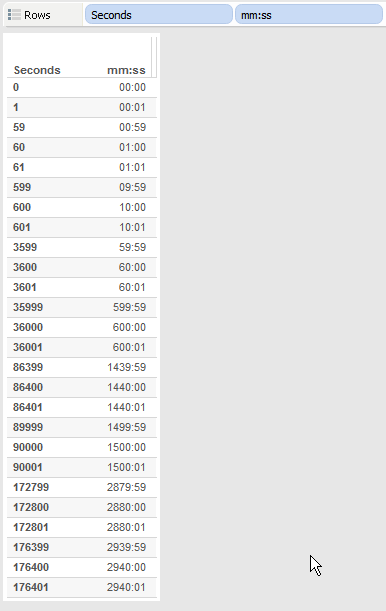For hh:mm:ss the formula gets a little more complex. Just as the seconds had to be transformed to make 0-59 seconds, minutes have to do a similar transformation:

```//replace [Seconds] with whatever field has the number of seconds in it
//and use a custom number format of 00:00:00 (drop the first 0 to get rid of leading 0's for hours)
IIF([Seconds] % 60 == 60,0,[Seconds] % 60)// seconds
+ IIF(INT([Seconds]/60) %60 == 60, 0, INT([Seconds]/60) %60) * 100 //minutes
+ INT([Seconds]/3600) * 10000 //hours```

And for dd:hh:mm:ss there’s yet another transformation to convert hours to 0-23:

```//replace [Seconds] with whatever field has the number of seconds in it
//and use a custom number format of 00:00:00:00 (drop the first 0 to get rid of leading 0's for days)
IIF([Seconds] % 60 == 60,0,[Seconds] % 60)// seconds
+ IIF(INT([Seconds]/60) %60 == 60, 0, INT([Seconds]/60) %60) * 100 //minutes
+ IIF(INT([Seconds]/3600) % 24 == 0, 0, INT([Seconds]/3600) % 24) * 10000 //hours
+ INT([Seconds]/86400) * 1000000 // days```

Here’s a view showing all three calculations: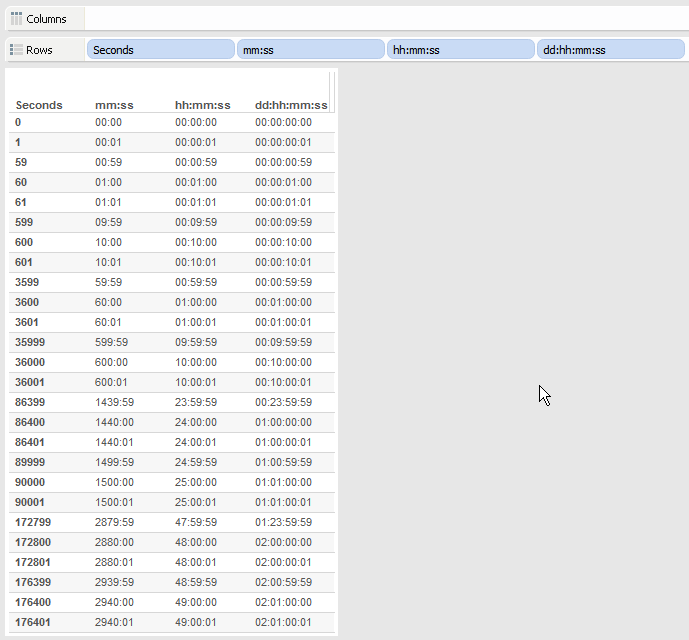A little dose of math, sprinkle some custom number formatting on it, and voila, there’s some usable duration formatting. If you wanted to keep going into weeks then there would need to be another level of calculation, to get into months and years I’d probably use a different approach because the intervals (month lengths and year lengths) aren’t fixed.

Here’s the workbook on Tableau Public: Duration Formatting

If this is useful to you, or you have an alternative technique, let me know in the comments below!

# Addendum on Aggregation (July 2015)

Some people people using this technique have run into problems as seen in the comments below and on the Tableau forums. For example, if we build a view like this and sum up all the values of Seconds with the duration calc applied (see the SUM() aggregation) the numbers don’t add up. In this view the total time in mm:ss should be 44558:00 but it’s showing up as 44554:80, a non-sensical amount: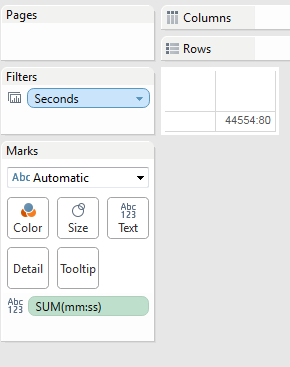Alternatively, if we keep Seconds in the view as a dimension but add a Grand Total, the Grand Total isn’t adding up either:The issue is with regards to order of operations. When we build the views with the grand total or by summing the measure, there’s an aggregation happening and it’s not happening at the right time vis-a-vis the formatting. I put together a series of graphics to explain what is going on.

In the original post the duration calculations are performed for each record and the results are treturned from the view. So it looks like this: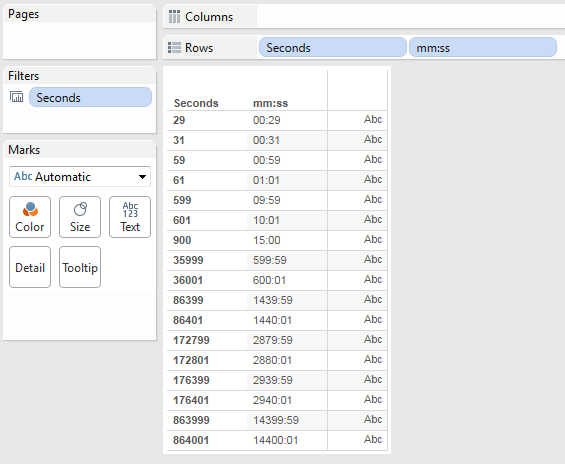So in this case here’s what’s going on under the hood: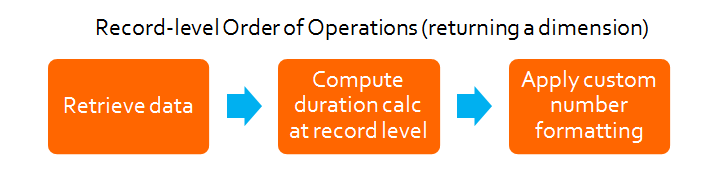That’s not often used in practice, more often a view looks like this, where the mm:ss is being used as a measure and then when the original calculation is brought into the view we can see that Tableau is actually aggregating the duration calculation with the default aggregation of SUM():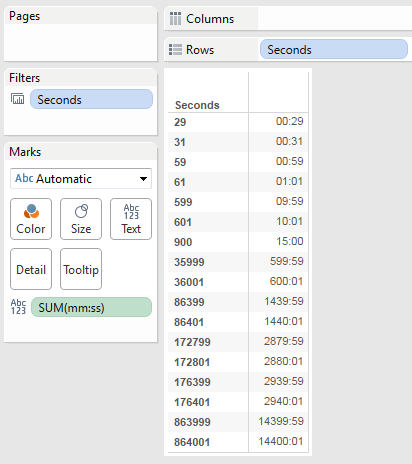However, that SUM() has no effect because the level of detail (the granularity) of the view is the same is the same as the data — in other words, the view is effectively displaying the raw data. So the order of operations is actually like this:So even though these two views look exactly the same, under the hood they are using two different ways to get there. Where this causes problems is when we apply an aggregation that is across multiple records or in a grand total or subtotal. The duration calculation that was meant to be applied to the final result is being applied to every record and it’s that transmogrified amount that is getting summed: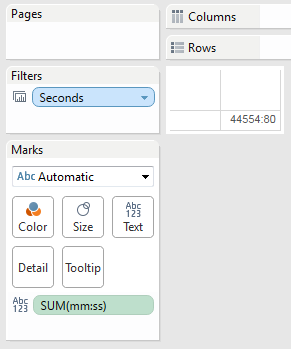The solution is pretty straightforward, we just need to do the aggregation *before* we apply the duration calculation. So instead of the [Seconds] of the original calculation we use Sum Seconds with the formula SUM([Seconds]), and the formula for mm:ss (agg) is:

```//replace [Sum Seconds] with whatever field has the number of seconds in it
IIF([Sum Seconds] % 60 == 60,0,[Sum Seconds] % 60)// seconds
+ INT([Sum Seconds]/60) * 100 //minutes```

So the order of operations is now:And here’s a view showing the inaccurate and now accurate aggregate calculations: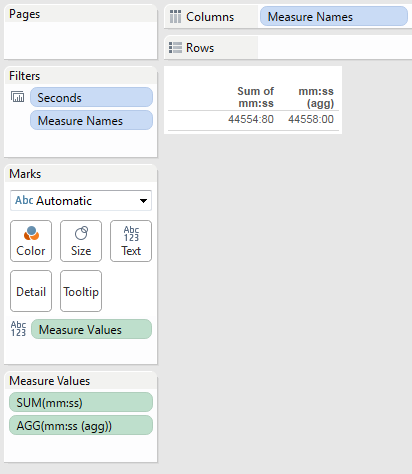And a grand total: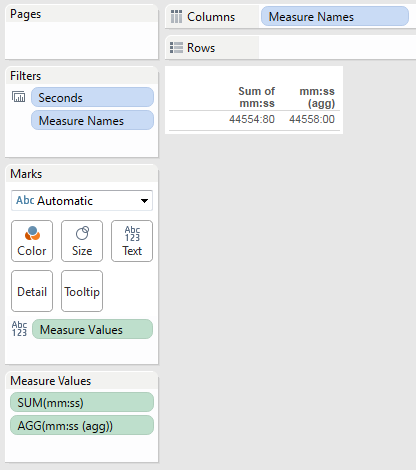I’ve updated the Duration Formatting workbook to reflect this addendum.

# Preparing Data

### DATE() and STR() functions behave differently depending on the data source (Excel/text/Access vs. extract)

http://community.tableausoftware.com/message/179624

DATEPARSE() for converting different date formats

DATEPARSE-ing Tips for Successful Speed Dating with Tableau 8.1

## Joining Vs. Blending

When one data source has subcategory & measures, and another has category & subcategory, can use Primary Groups to effectively create the category within the first data source. KB has article on this, search for “creating primary groups from a secondary source”.

### Data blending and getting aggregations right:

Workbook with three options (extract, control data relationships for blend, custom SQL) by Jeff Mills:
http://community.tableausoftware.com/message/183888#183888

Creating a Tableau Data Source .tds for connecting to just the database and not the table by Craig Bloodworth:
http://www.theinformationlab.co.uk/2012/11/26/connect-to-a-database-without-specifying-a-table-video/

Outer joining multiple Excel tables
https://www.interworks.com/blogs/tmccullough/2013/06/26/outer-joining-multiple-excel-tables-tableau-8

Custom datasource config files:
http://community.tableausoftware.com/message/227764

[loop category=”wikicontent” tag=”preparing,reshaping”]
[field title] – Added [field date]
[content]
[/loop]

### Related posts:

[loop tag=”preparing,reshaping,anonymizing,blinding,reshape,reshaper” exclude=”this” relation=”and” compare=”not” taxonomy=”category” value=”wikicontent”]